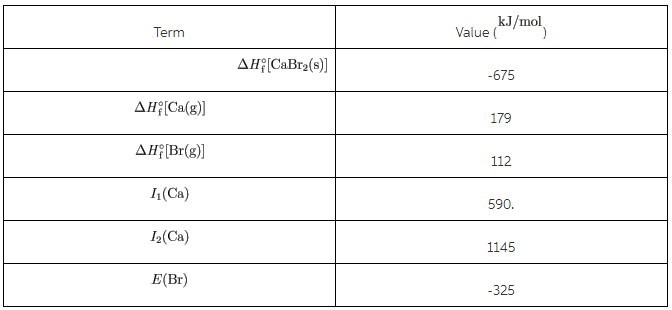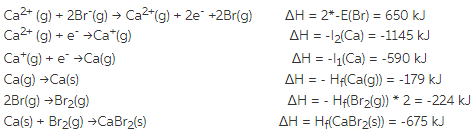# Given the following thermodynamic data,calculate the lattice energy of CaBr2(s)

Given the following thermodynamic data,calculate the lattice energy of CaBr2(s).You are also given information on the Born-Harber Cycle which isbelow